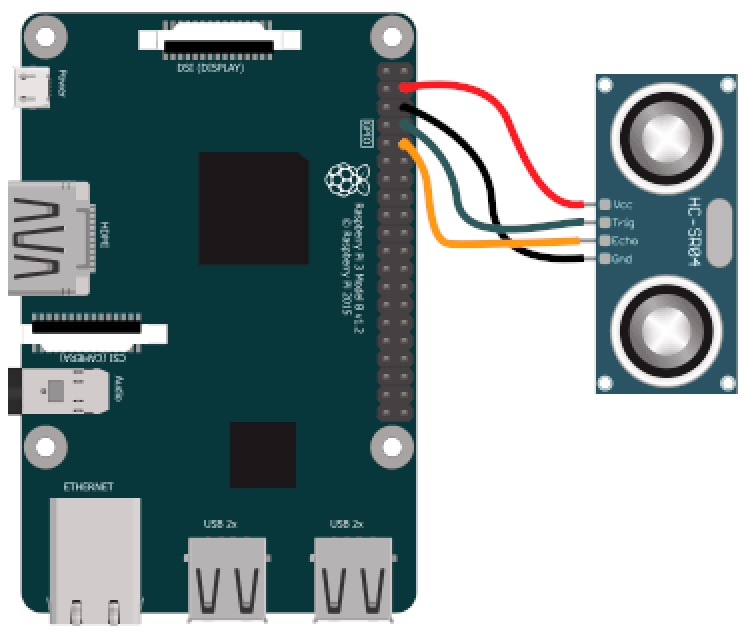The SR04 module is a popular ultrasonic distance sensor that is commonly used with microcontrollers like Arduino and Raspberry Pi. It can be used to measure distances from 2cm to 400cm with an accuracy of 0.3cm. In this blog post, we will explore how to use the SR04 module with a Raspberry Pi.

## HC-SR04 Module

The HC-SR04 module consists of a transmitter and receiver, and works by sending out a high-frequency sound pulse and timing how long it takes for the pulse to bounce back off an object and return to the sensor. By knowing the speed of sound and the time it takes for the pulse to return, the module can calculate the distance between itself and the object.

## Materials

Before starting the project, you will need to gather the following materials:

## Making the Connections

The SR04 sensor has four pins: VCC, GND, Trig, and Echo. The VCC and GND pins are used to provide power to the sensor. The Trig pin is used to send the ultrasonic pulse, and the Echo pin is used to receive the reflected signal. In this tutorial we will use GPIO14 (board pin 8) for the TRIG pin and GPIO15 (board pin 10) for the ECHO as seen in this diagram:## Writing the Python code

With the SR04 module connected and the required packages installed, we're ready to write a Python script to read the distance measurements from the sensor.

``````import RPi.GPIO as GPIO
import time

GPIO.setmode(GPIO.BOARD)

trig_pin = 8  # GPIO14 pin connected to the Trig pin on SR04 module
echo_pin = 10  # GPIO15 pin connected to the Echo pin on SR04 module

GPIO.setup(trig_pin, GPIO.OUT)
GPIO.setup(echo_pin, GPIO.IN)

def distance():
# Send a 10us pulse to trigger the SR04 module
GPIO.output(trig_pin, True)
time.sleep(0.00001)
GPIO.output(trig_pin, False)

# Measure the duration of the pulse from the Echo pin
start_time = time.time()
while GPIO.input(echo_pin) == 0:
start_time = time.time()

end_time = time.time()
while GPIO.input(echo_pin) == 1:
end_time = time.time()

# Calculate the distance based on the duration of the pulse
duration = end_time - start_time
distance = duration * 17150  # speed of sound in cm/s
distance = round(distance, 2)  # round to two decimal places

return distance

# Main loop
try:
while True:
dist = distance()
print(f"Distance: {dist} cm")
time.sleep(1)

except KeyboardInterrupt:
GPIO.cleanup()``````
1. We first import the RPi.GPIO library and the time module. We also set the GPIO mode to `BOARD`, which means we refer to the GPIO pins by their physical pin numbers on the Pi board.
2. We define the GPIO pins connected to the Trig and Echo pins on the SR04 module.
3. We set up the Trig pin as an output pin and the Echo pin as an input pin.
4. We define a function `distance()` that sends a 10us pulse to the Trig pin to trigger the SR04 module. It then measures the duration of the pulse from the Echo pin and calculates the distance based on the speed of sound.
5. In the main loop, we call the `distance()` function and print the distance to the console. We also add a delay of 1 second between measurements.
6. We handle the `KeyboardInterrupt` exception by cleaning up the GPIO pins.

## Run the code

After running your file you should see the distance measurements printed to the console every second. Note that you may need to adjust the GPIO pin numbers to match the pins you have connected to the SR04 module on your Pi.

## Conclusion

In this tutorial, we learned how to leverage an SR04 sonar sensor module using a Raspberry Pi and control it using Python code. We hope this tutorial was helpful and inspires you to build your own robotics projects using Raspberry Pi.

Good luck and happy coding!